### Home > PC > Chapter 4 > Lesson 4.1.1 > Problem4-8

4-8.
1. Given the equation s2 + c2 = 1, simplify each of the following expressions. Homework Help ✎

1. c2 + s2

2. 1 − c2

3. 1 − s2

4. s + c

5. 4(1 − s2)

6. 4 − 4s2Rewrite the given equation in its various forms.
Substitute the appropriate expressions in the problems below.

s2 = 1 − c2
c2 = 1 − s2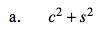This is the same as: s2 + c2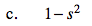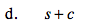This cannot be further simplified.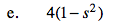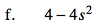Factor out a 4 first. Then substitute an expression for s2.